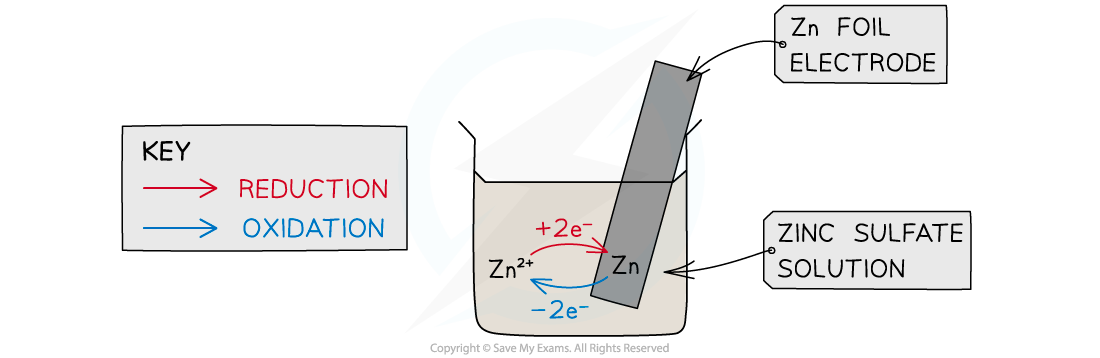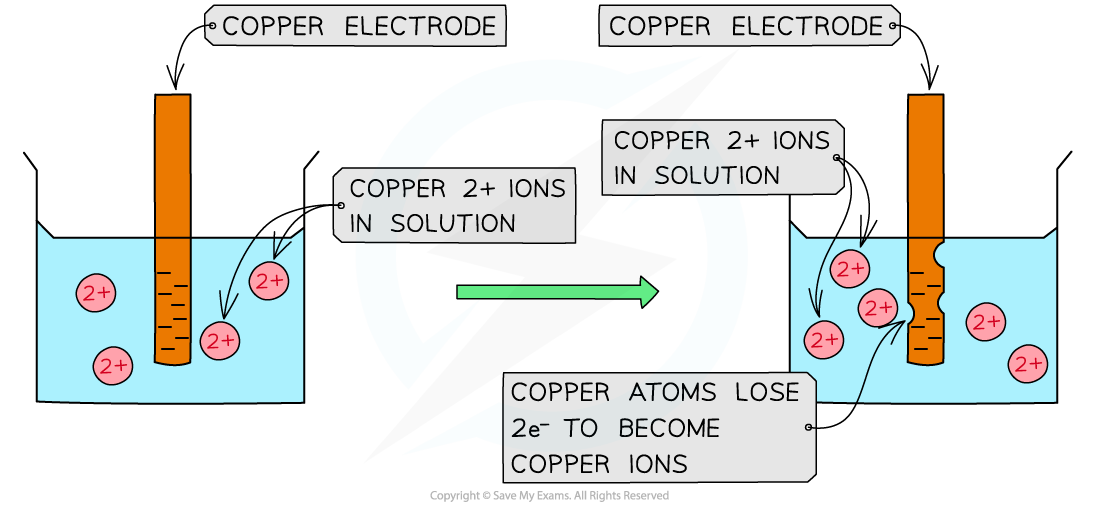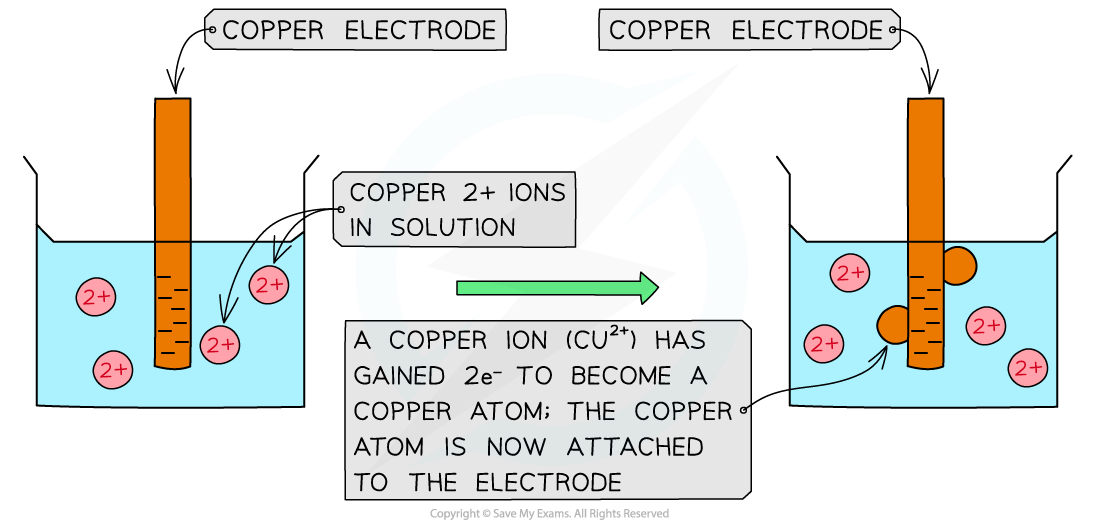# AQA A Level Chemistry复习笔记5.4.1 Representing Cells

### Representing Cells

• Electrochemical cells generate electricity from spontaneous redox reactions
• For example:

Zn (s)  + CuSO4 (aq)→ Cu (s)  + ZnSO4 (aq)

• Instead of electrons being transferred directly from the zinc to the copper ions, a cell is built which separates the two redox processes
• For example:

Zn (s)  ⇌  Zn2+ (aq) + 2e–

• If a rod of metal is dipped into a solution of its own ions, an equilibrium is set up
• Each part of the cell is called a half cellWhen a metal is dipped into a solution containing its ions an equilibrium is established between the metal and its ions. This is the basis of a half cell in an electrochemical cell.

• This is a half cell and the strip of metal is an electrode
• The position of the equilibrium determines the potential difference between the metal strip and the solution of metal
• The Zn atoms on the rod can deposit two electrons on the rod and move into solution as Zn2+ ions:

Zn(s) ⇌ Zn2+(aq) + 2e–

• This process would result in an accumulation of negative charge on the zinc rod
• Alternatively, the Zn2+ ions in solution could accept two electrons from the rod and move onto the rod to become Zn atoms:

Zn2+(aq) + 2e–  ⇌ Zn(s)

• This process would result in an accumulation of positive charge on the zinc rod
• In both cases, a potential difference is set up between the rod and the solution
• This is known as an electrode potential
• A similar electrode potential is set up if a copper rod is immersed in a solution containing copper ions (e.g. CuSO4), due to the following processes:

Cu2+(aq) + 2e–  ⇌ Cu(s)   – reduction (rod becomes positive)

Cu(s) ⇌ Cu2+(aq) + 2e–    – oxidation (rod becomes negative)Oxidation of copper atomsReduction of copper(II) ions

• Note that a chemical reaction is not taking place – there is simply a potential difference between the rod and the solution
• The potential difference will depend on
• the nature of the ions in solution
• the concentration of the ions in solution
• the type of electrode used
• the temperature

#### Electrode potential

• The electrode (reduction) potential (E) is a value which shows how easily a substance is reduced
• These are demonstrated using reversible half equations
• This is because there is a redox equilibrium between two related species that are in different oxidation states

• When writing half equations for this topic, the electrons will always be written on the left-hand side (demonstrating reduction)
• The position of equilibrium is different for different species, which is why different species will have different electrode (reduction) potentials
• The more positive (or less negative) an electrode potential, the more likely it is for that species to undergo reduction
• The equilibrium position lies more to the right

• For example, the positive electrode potential of bromine below, suggests that it is likely to get reduced and form bromide (Br-) ions

Br2(l) + 2e- ⇌ 2Br-(aq)        E = +1.09 V

• The more negative (or less positive) the electrode potential, the less likely it is that reduction of that species will occur
• The equilibrium position lies more to the left

• For example, the negative electrode potential of sodium suggests that it is unlikely that the sodium (Na+) ions will be reduced to sodium (Na) atoms

Na+(aq) + e- ⇌ Na(s)        E = -2.71 V

#### Conventional Representation of Cells

• Chemists use a type of shorthand convention to represent electrochemical cells
• In this convention:
• A solid vertical (or slanted) line shows a phase boundary, that is an interface between a solid and a solution
• A double vertical line (sometimes shown as dashed vertical lines) represents a salt bridge
• A salt bridge has mobile ions that complete the circuit
• Potassium chloride and potassium nitrate are commonly used to make the salt bridge as chlorides and nitrates are usually soluble
• This should ensure that no precipitates form which can affect the equilibrium position of the half cells

• The substance with the highest oxidation state in each half cell is drawn next to the salt bridge
• The cell potential difference is shown with the polarity of the right hand electrode

• The cell convention for the zinc and copper cell would be

Zn (s)∣Zn2+ (aq) ∥Cu2+ (aq)∣Cu (s)                  E cell = +1.10 V

• This tells us the copper half cell is more positive than the zinc half cell, so that electrons would flow from the zinc to the copper
• The same cell can be written as:

Cu (s)∣Cu2+ (aq) ∥Zn2+ (aq)∣Zn (s)                  E cell = -1.10 V

• The polarity of the right hand half cell is negative, so we can still tell that electrons flow from the zinc to the copper half cell

#### Worked Example

Writing a cell diagram

If you connect an aluminium electrode to a zinc electrode, the voltmeter reads 0.94V and the aluminium is the negative. Write the conventional cell diagram to the reaction.

Al (s)∣Al3+ (aq) ∥ Zn2+ (aq)∣Zn (s)                  E cell = +0.94 V

It is also acceptable to include phase boundaries on the outside of cells as well:

∣ Al (s)∣Al3+ (aq) ∥ Zn2+ (aq)∣Zn (s) ∣                  E cell = +0.94 V

#### Exam Tip

Students often confuse the redox processes that take place in electrochemical cells.

• Oxidation takes place at the negative electrode.
• Reduction takes place at the positive electrode.

Remember, oxidation is the loss of electrons, so you are losing electrons at the negative.

∣ Al (s)∣Al3+ (aq) ∥Zn2+ (aq)∣Zn (s) ∣                  Ecell = +0.94 V